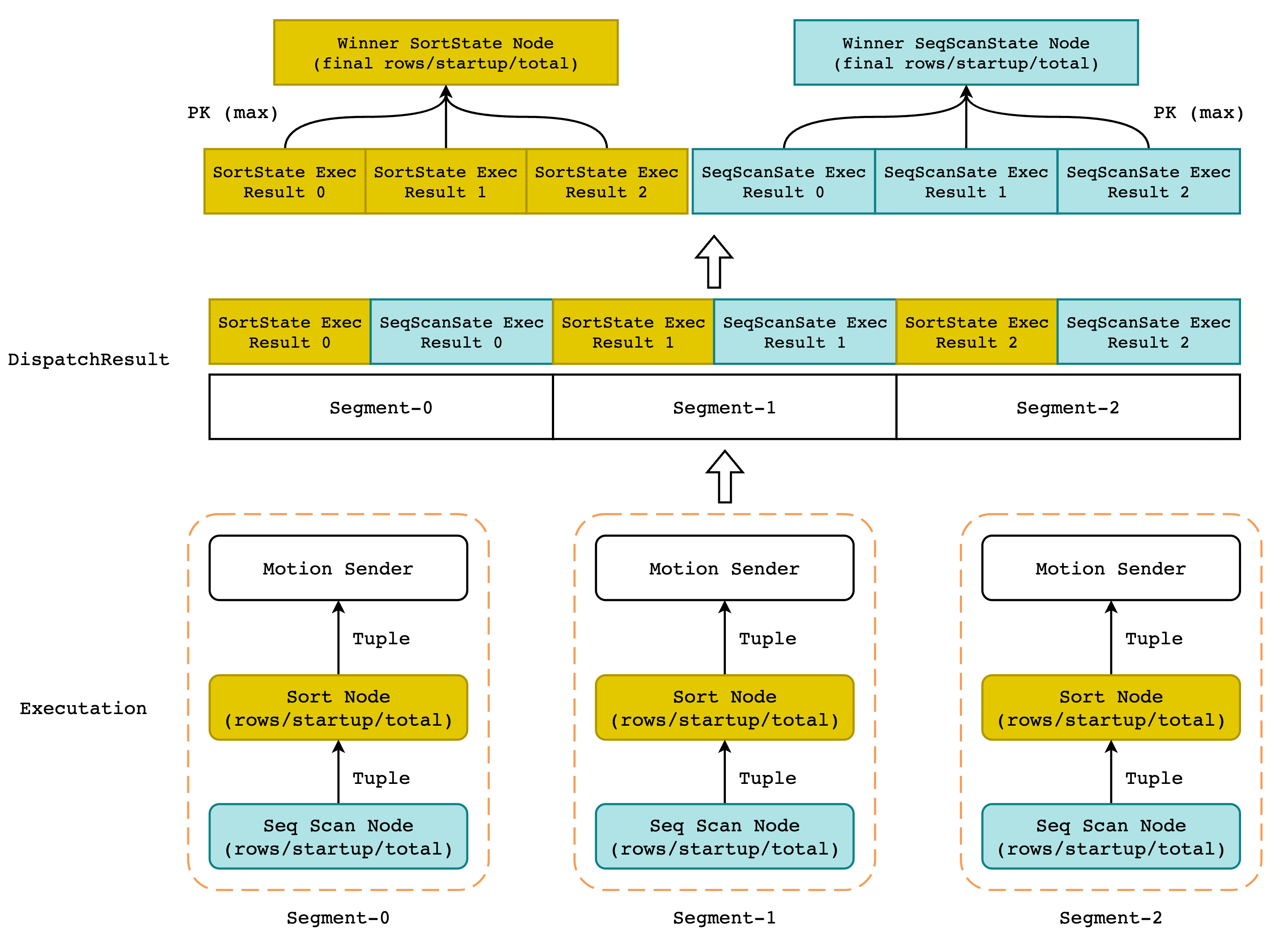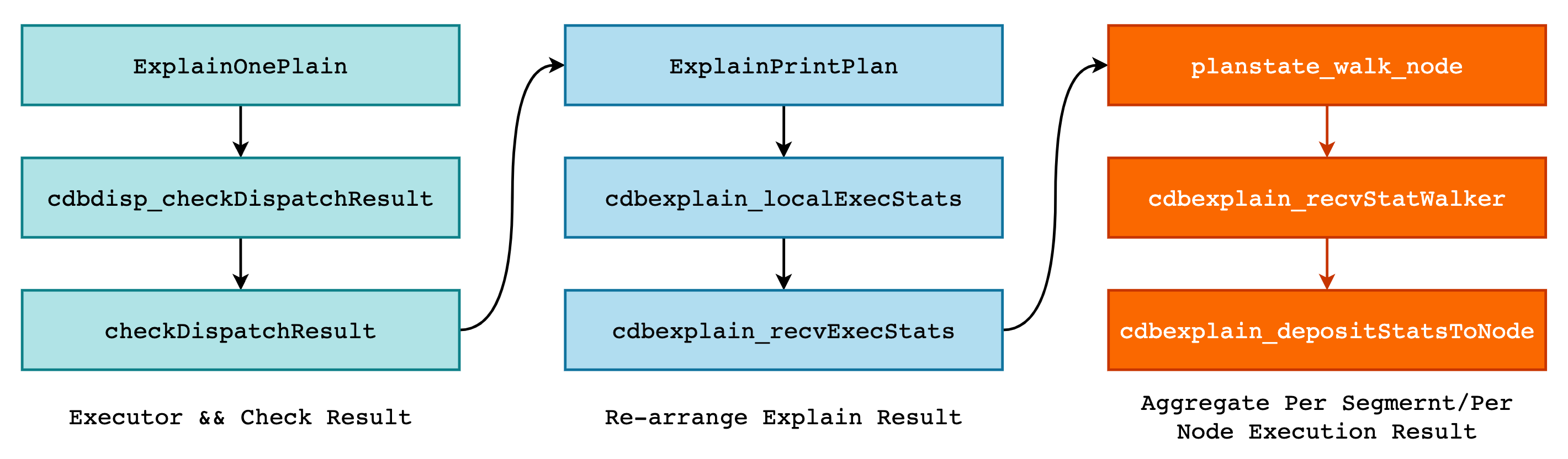### 1. Greenplum 中的 EXPLAIN ANALYZE

``````postgres=# create table t (a int, b int);
postgres=# insert into t select random() * 1000, random() * 1000 from generate_series(1, 1000000);

postgres=# explain (costs off, analyze) select * from t where a > 500 order by a;
QUERY PLAN
--------------------------------------------------------------------------------------------------------------------
Gather Motion 3:1  (slice1; segments: 3) (actual time=135.091..360.139 rows=498802 loops=1)
Merge Key: a
->  Sort (actual time=134.412..173.091 rows=177922 loops=1)
Sort Key: a
Sort Method:  quicksort  Memory: 91424kB
->  Seq Scan on t (actual time=0.035..70.378 rows=177922 loops=1)
Filter: (a > 500)
Rows Removed by Filter: 162816
Planning Time: 0.270 ms
(slice0)    Executor memory: 67K bytes.
(slice1)    Executor memory: 11341K bytes avg x 3 workers, 11706K bytes max (seg2).  Work_mem: 11706K bytes max.
Memory used:  129024kB
Optimizer: Postgres query optimizer
Execution Time: 381.492 ms
(14 rows)
``````

actual time: actual time 表示当前执行节点的实际执行情况，其表现形式为 start…total。其中 start 表示该节点的实际启动时间，也就是当前节点向上层节点返回第一条 tuple 的准备时间，total 则是该节点的执行总时间，单位均为毫秒。

rows: 当前节点总计向上层节点返回的 tuple 数量，而不是当前节点实际处理的 tuple 数量。以 Seq Scan 节点为例，过滤条件一共过滤了 162816 条数据，向 Sort 节点返回了 177922 条数据，即 Seq Scan 节点一共扫描了 162816 + 177922 = 340738 条数据。结合 actual time 来看，Seq Scan 节点处理 340738 条数据一共耗时 70ms。

loops: loops 表示当前节点的执行次数，比如在 Nested Loop 中，内表可能会被扫描多次，那么此时 loops 就表示实际的扫描次数，同时 actual time 和 rows 的输出结果为每一次执行的平均值。

``````postgres=# explain (costs off, analyze) select *
from foo, bar where foo.a = bar.a and foo.a < 50;

QUERY PLAN
------------------------------------------------------------------------------------------
Gather Motion 3:1  (slice1; segments: 3) (actual time=1.472..5.065 rows=4830 loops=1)
->  Nested Loop (actual time=0.240..3.381 rows=1927 loops=1)
->  Bitmap Heap Scan on bar (actual time=0.220..0.518 rows=187 loops=1)
Recheck Cond: (a < 50)
->  Bitmap Index Scan on bar_a (actual time=0.190..0.190 rows=187 loops=1)
Index Cond: (a < 50)
->  Index Scan using foo_a on foo (actual time=0.000..0.011 rows=10 loops=187)
Index Cond: ((a = bar.a) AND (a < 50))
Planning Time: 1.333 ms
(slice0)    Executor memory: 80K bytes.
(slice1)    Executor memory: 2408K bytes avg x 3 workers, 2408K bytes max (seg0).
Memory used:  129024kB
Optimizer: Postgres query optimizer
Execution Time: 6.288 ms
``````

Buckets && Batches: TODO

Slice information: TODO

Memory used: Memory used 表示在 Greenplum 的内存资源管理下，为该查询预先分配的内存数量，由函数 `ResourceManagerGetQueryMemoryLimit()` 完成，并不表示实际的内存使用。

``````postgres=# explain (costs off, analyze) select * from t where a > 500 order by a;
QUERY PLAN
-----------------------------------------------------------------------------------------------
Gather Motion 3:1  (slice1; segments: 3) (actual time=278.764..707.422 rows=997604 loops=1)
Merge Key: a
->  Sort (actual time=278.024..360.312 rows=355844 loops=1)
Sort Key: a
Sort Method:  quicksort  Memory: 182846kB
->  Seq Scan on t (actual time=0.037..140.474 rows=355844 loops=1)
Filter: (a > 500)
Rows Removed by Filter: 325632
Memory used:  129024kB
Optimizer: Postgres query optimizer
Execution Time: 747.208 ms
``````

## 2. EXPLAIN ANALYZE 的实现“缝合怪”，我觉得这是一个形容 EXPLAIN ANALYZE 结果的最佳词语。其原因在于 EXPLAIN ANALYZE 结果中的每一个执行节点（例如 Hash Join、Sort、Seq Scan、Index Scan） 都来自所有 segments 执行结果中 tuple 数量最多的结果节点，听起来有点儿绕，如下图所示:``````typedef struct
{
double		vmax;			/* 统计数据的最大值 */
double		vsum;			/* 统计数据的和 */
int			vcnt;			/* 统计数据的个数，和 vsum 可计算平均值 */
int			imax;			/* 拥有最大值的 segment index */
} CdbExplain_Agg;

typedef struct CdbExplain_DepStatAcc
{
CdbExplain_Agg agg;                /* 数据统计信息 */

CdbExplain_StatHdr  *rshmax;       /* 最大值所在的原始 QD 结果数组 */
CdbExplain_StatInst *rsimax;       /* 指向 rshmax 中具体的 nstrumentation 对象*/
CdbExplain_StatInst *nsimax;       /* 指向 node summary 中具体的 instr 对象*/

double		max_total;                /* 执行当前节点的最长总时间 */
instr_time	firststart_of_max_total;  /* 与 max_total 对应的实际启动时间 */
} CdbExplain_DepStatAcc;
``````

`ntuples` 为例，在遍历 Diapatch Results 数组时更新对应的 `CdbExplain_Agg` 和其它字段即可:

``````CdbExplain_DepStatAcc ntuples;
cdbexplain_depStatAcc_init0(&ntuples);

for (imsgptr = 0; imsgptr < ctx->nmsgptr; imsgptr++)
{
rsh = ctx->msgptrs[imsgptr];        /* 获取第 imsgptr 个 QE 的执行结果 */
rsi = &rsh->inst[ctx->iStatInst];   /* 获取具体的 node 结果 */

nsi = &ns->insts[rsh->segindex - ns->segindex0];
*nsi = *rsi;

/* 在该函数中使用 rsi 中的信息更新统计信息 */
cdbexplain_depStatAcc_upd(&ntuples, rsi->ntuples, rsh, rsi, nsi);
}
``````

`cdbexplain_depStatAcc_upd()` 的实现也非常简单:

``````static inline bool cdbexplain_agg_upd(CdbExplain_Agg *agg, double v, int id)
{
if (v > 0)
{
agg->vsum += v;     /* 更新累加值 */
agg->vcnt++;        /* 更新计数器 */

if (v > agg->vmax || agg->vcnt == 0)
{
agg->vmax = v;  /* 更新最大值以及最大值对应的 index */
agg->imax = id;
return true;
}
}
return false;
}

static inline void
cdbexplain_depStatAcc_upd(CdbExplain_DepStatAcc *acc,
double v,
CdbExplain_StatHdr *rsh,
CdbExplain_StatInst *rsi,
CdbExplain_StatInst *nsi)
{
if (cdbexplain_agg_upd(&acc->agg, v, rsh->segindex))
{
acc->rshmax = rsh;
acc->rsimax = rsi;
acc->nsimax = nsi;
}

/* 更新 total 以及 firststart_of_max_total */
if (acc->max_total < nsi->total)
{
acc->max_total = nsi->total;
INSTR_TIME_ASSIGN(acc->firststart_of_max_total, nsi->firststart);
}
}
``````

Greenplum 为了防止 Filter 节点将所有的元组都过滤掉，使得所有 segment 的 rows 等于 0，从而导致 actual time 中的 start 和 total 为 0 的情况出现，Greenplum 将会在此时将 start 和 total 替换成最长的执行时间，而不是返回 tuple 数量最多的节点的执行时间。